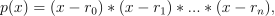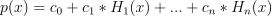/NumPy 1.17

# numpy.polynomial.hermite.hermfromroots

`numpy.polynomial.hermite.hermfromroots(roots)` [source]

Generate a Hermite series with given roots.

The function returns the coefficients of the polynomialin Hermite form, where the `r_n` are the roots specified in `roots`. If a zero has multiplicity n, then it must appear in `roots` n times. For instance, if 2 is a root of multiplicity three and 3 is a root of multiplicity 2, then `roots` looks something like [2, 2, 2, 3, 3]. The roots can appear in any order.

If the returned coefficients are `c`, thenThe coefficient of the last term is not generally 1 for monic polynomials in Hermite form.

Parameters: `roots : array_like` Sequence containing the roots. `out : ndarray` 1-D array of coefficients. If all roots are real then `out` is a real array, if some of the roots are complex, then `out` is complex even if all the coefficients in the result are real (see Examples below).

See also

`polyfromroots`, `legfromroots`, `lagfromroots`, `chebfromroots`, `hermefromroots`

#### Examples

```>>> from numpy.polynomial.hermite import hermfromroots, hermval
>>> coef = hermfromroots((-1, 0, 1))
>>> hermval((-1, 0, 1), coef)
array([0.,  0.,  0.])
>>> coef = hermfromroots((-1j, 1j))
>>> hermval((-1j, 1j), coef)
array([0.+0.j, 0.+0.j])
```

© 2005–2019 NumPy Developers
Licensed under the 3-clause BSD License.
https://docs.scipy.org/doc/numpy-1.17.0/reference/generated/numpy.polynomial.hermite.hermfromroots.html